# The Magnetic Tower of Hanoi

 Table 1: Minimum number of disk-moves required to solve the classical Tower of Hanoi puzzle. N is the total number of disks participating in the game and k is the disk number in the ordered stack, counting from bottom to top. The k-th disk "makes" 2(k-1) moves (Equation 1). The total number of disk-moves required to solve an N-disk puzzle is 2N – 1 (Equation 2). Table 1 clealy shows how (elegantly) the classical ToH spans base 2. Let's see now how base 3 is spanned by the far more intricate Magnetic Tower of Hanoi puzzle. In the Magnetic Tower of Hanoi puzzle, we still use three posts and N disks. However, the disk itself, the move definition and the game rules are all modified (extended). The rigorous description of the MToH puzzle is as follows: Puzzle Components: Three equal posts A set of N different-diameter disks Each disk's "bottom" surface is colored Blue and its "top" surface is colored Red Puzzle-start setting: N disks arranged in a bottom-to-top descending-size order on a "Source" Post (Figure 2) The Red surface of every disk in the stack is facing upwards (Figure 2). Note that the puzzle-start setting satisfies the "Magnet Rule" (see below). And needless to say, Red is chosen arbitrarily without limiting the generality of the discussion. Move: Lift a disk off one post Turn the disk upside down and land it on another post Disk-placement rules: The Size Rule: A small disk can not "carry" a larger one (Never land a large disk on a smaller one) The Magnet Rule: Rejection occurs between two equal colors (Never land a disk such that its bottom surface will touch a co-colored top surface of the "resident" disk) Puzzle-end state: N disks arranged in a bottom-to-top descending-size order on a "Destination" Post (one of the two originally-free posts)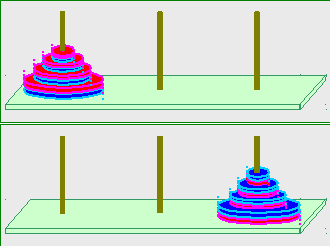Figure 2: The Magnetic Tower of Hanoi puzzle. Top – puzzle-start setting. The puzzle consists of three posts, and N two-color disks. The puzzle solution process ("game") calls for one-by-one disk moves restricted by two rules – the Size Rule and the Magnet Rule. The puzzle is solved when all disks are transferred from a "Source" Post to a "Destination" Post - bottom. Given the above description of the MToH puzzle, let's calculate the number of moves necessary to solve the puzzle. We start by explicitly solving the N=1, N=2 and N=3 cases. Explicit solution for the first three stacks of the MToH puzzle The N = 1 case is trivial – move the disk from the Source Post to a Destination Post (Figure 3).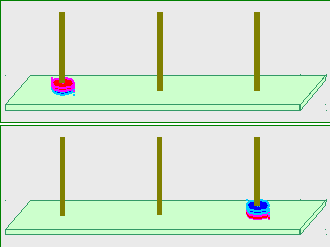Figure 3: The start-setting (top) and the end-state (bottom) for the N=1 MToH puzzle. The number of moves required to solve the puzzle is P(1) = 1. Thus, for the N=1 case we have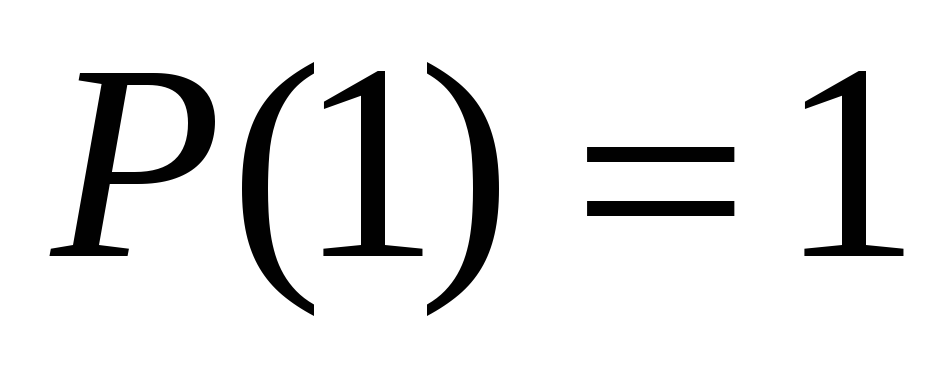;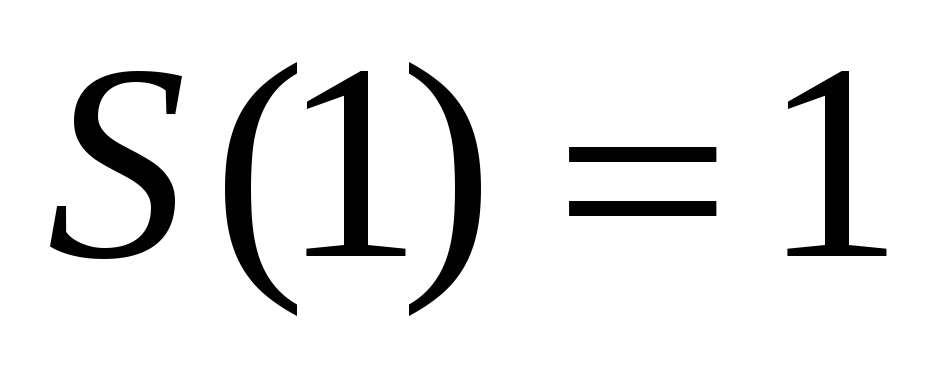. (3) Let's see the N=2 case.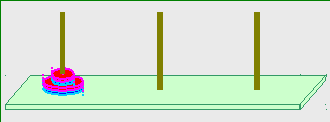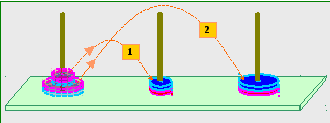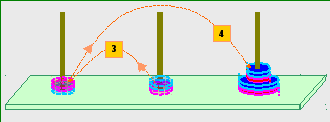Share with your friends: